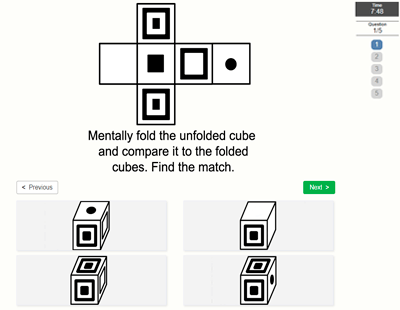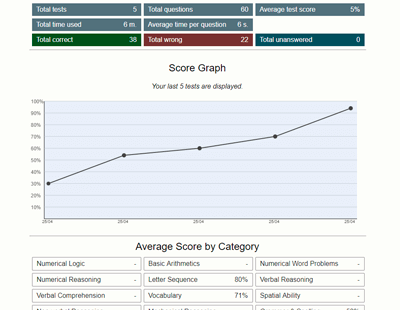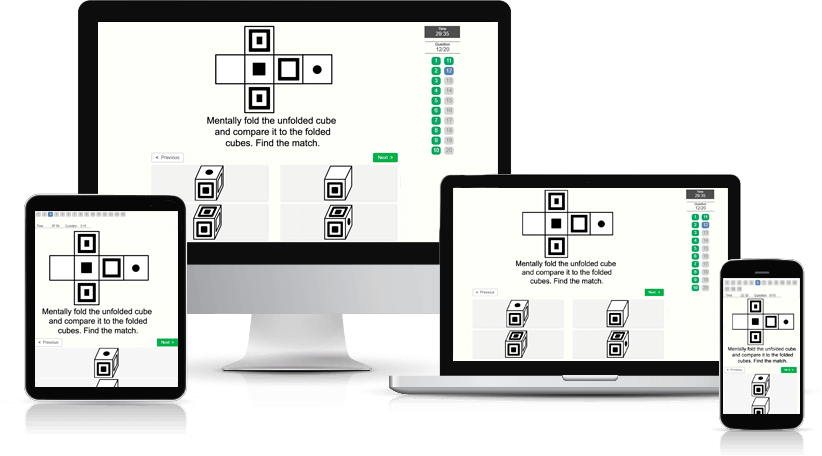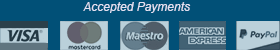﻿ Non-verbal Reasoning Test - Aptitude-Test.com

Non-verbal Reasoning Test

Spatial ability is the capacity to understand and remember the spatial relations among objects. In a spatial ability test (also called spatial reasoning test) you are required to mentally manipulate 2-dimensional and 3-dimensional figures.

What questions can I expect?
The questions found in a spatial ability test are often of a similar nature. Here's a list of the most common question types:

• Cubes: You are given a flat (2-dimensional) pattern which can be folded into a cube. Between a number of 3-dimensional cubes you are to choose the one that the pattern can be folded into.
• Paper folding: In the question you are shown a paper being folded in steps. A hole is then punched one or more places through the folded paper. You are to find the answer option that correctly shows how the paper would look after being unfolded again.
• 2-d rotating figures: The question image is a drawn figure of some type. You are to find the figure that, after being rotated is exactly the same as the figure in the question image.
• 3-d rotating objects: The question image is a 3-dimensional object. You are to find the object that, after being rotated is exactly the same as the object in the question image.
• Mirroring: You are given a drawn figure in the question. You are now to find the answer that is a mirror image of the question figure. Mirroring can be both vertically and horizontally.
• Assembling objects: You are given four or more parts of an object. You now have to find the object that can be built with the object parts. These questions can be both 3-dimensional and 2-dimensional.

Learn more about some of the question types by trying our free test. Get a Test Prep Account to practice on all of the question types mentioned above, and more, to improve your spatial ability.

Where are Non-verbal Reasoning tests used?
Spatial ability tests are used as part of the recruitment process of engineers, architects, chemists, mechanics, line assembly workers and more. The tests are often a part of the school admission exam for educations as architect, graphic designer, air traffic controller, pilot, and more.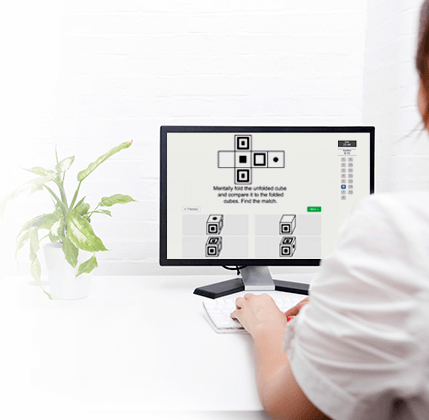Practice Non-verbal Reasoning Test

Try a free Non-verbal Reasoning Test.
This free practice test contains 6 test questions and has a time limit of 4 minutes.

Would you like to improve your test score? Practice smart with a Test Prep Account.

Practice on 141 Non-verbal Reasoning test questions and a total of 439 non-verbal aptitude test questions with detailed description and score statistics.

The many features of a Test Prep Account:

• Preparation software developed under a didactic and methodical perspective
• High-quality practice test questions
• Clearly explained solutions
• Accessibile on all devices
• Detailed score data and progression charts
• Reference scores to compare your performance against others
• Training Assistant
• Practice mode (feedback after every question and no time limit)